Home > CC4 > Chapter A > Lesson A.1.3 > ProblemA-31

A-31.

Simplify the following expressions by combining like terms, if possible. Algebra tiles may be helpful. Homework Help ✎

1. x + x − 3 + 4x2 + 2xx

2. 8x2 + 3x − 13x2 + 10x2 − 25xx

3. 4x + 3y

4. 20 + 3xy − 3 + 4y2 + 10 − 2y2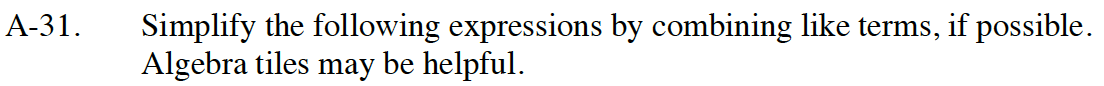Rearrange and put like terms together.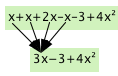Organize the terms from the greatest to the smallest power.

4x² + 3x − 3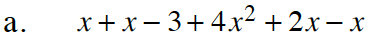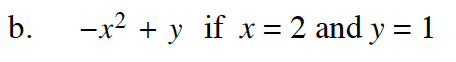Follow the steps in part (a).

5x² − 23x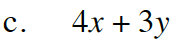Are there any like terms to combine?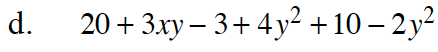Follow the steps in part (a).